### MS 095 PreAlgebra Quiz 02 • Name: __________

Matching

1. Given:
marble: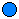pompom: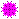red yarn circle: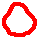Solve the following equation:+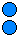=+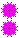2. __________ Find the volume of a box of Lux® soap with a length of 10cm, a width of 3cm, and a height of 8cm.
3. __________ Find the volume of a cylindrical tire with a radius of 50 centimeters and a length of 30 centimeters.
4. __________ Find the volume of a tennis ball with a radius of 325mm.
5. __________ What is 0 ÷ 0 equal to?
6. __________ What is –172 ÷ 0 equal to?
7. ____________________ Multiply out: (2p – 1)(2p + 1)
8. __________ Can an even divided by an odd ever be a whole number?
9. __________ Where the result is a whole number, the result of an odd divided by an odd is:
1. even
2. odd
3. either even or odd
4. neither even nor odd
10. __________ Find Ö512.
11. __________ Solve for x: –5x + 15 = –75
12. Write 3x + 7 = –5 in yarn | marble | pompom notation.